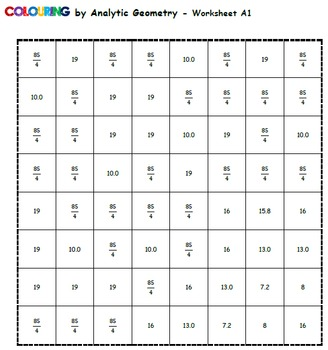# Analytic Geometry... Under the Sea! (Collaborative Class Colouring Task)9th - 11th
Subjects
Standards
Resource Type
Formats Included
• Zip
Pages
45 pages

### Description

Engage your whole class in a fun assignment that combines collaborative motivation with individual accountability. All 20 worksheets combine to create the large mosaic image!

Each student's worksheet contains 12 questions that span a variety of linear analytic geometry topics. (see list below) I designed this activity as a culminating activity for my grade 10 academic class (MPM2D in the Ontario curriculum). I handed it out at the start of the unit and had them complete it in the background as the topics were taught.

Students are highly motivated by the idea of colouring! The collaborative aspect of the image is also a compelling part of these "Colouring by..." products.

Caluculate... Colour.... Cut... Combine!! (Then hang it up in your classroom because it will look awesome!)

Check out the product preview to view sample worksheets, teaching tips, and answer keys!

If you like this product, be sure the check out the whole Collaborative Math Mosaic directory, sorted by topic!

INCLUDED (.docx and .pdf in zip folder)
◾20 different linear analytic geometry worksheets / colouring pages that combine to create a giant mosaic image (the octopus)
◾Answer keys! See the completed mosaic, the answer range assigned to each colour (i.e. all questions for Blue have an answer between 3 and 7) AND the complete answer key for all questions on every worksheet.
◾Teaching Tips page
◾Problem Order summary, so you know the type of questions each sheet asks.

MATH INVOLVED
◾Calculate the midpoint of a line segment given its endpoints.
◾Given one endpoint and the midpoint of a line segment, determine the other endpoint coordinates.
◾Calculate the slope or length of medians for a given triangle.
◾calculate the slope or length of altitude segments for a given triangle.
◾calculate the slope of right bisector for a given a line segment.
◾y-intercept of the line passing through two given points
◾determine the perimeter of a triangle given the coordinates of its vertices
◾determine the shortest (perpendicular) distance from a point to a line

Specific order of problems on each worksheet:
1) midpoint of segment
2) midpoint of segment
3) slope of median
4) length of median
5) slope of right bisector
6) y-intercept from points
7) slope of altitude
8) length of segment
9) slope or intercept from standard form
10) length of altitude
11) perimeter of triangle
12) perpendicular distance from point to line
Total Pages
45 pages
Included
Teaching Duration
2 days
Report this Resource to TpT
Reported resources will be reviewed by our team. Report this resource to let us know if this resource violates TpT’s content guidelines.

### Standards

to see state-specific standards (only available in the US).
Prove the slope criteria for parallel and perpendicular lines and use them to solve geometric problems (e.g., find the equation of a line parallel or perpendicular to a given line that passes through a given point).
Find the point on a directed line segment between two given points that partitions the segment in a given ratio.
Use coordinates to compute perimeters of polygons and areas of triangles and rectangles, e.g., using the distance formula.